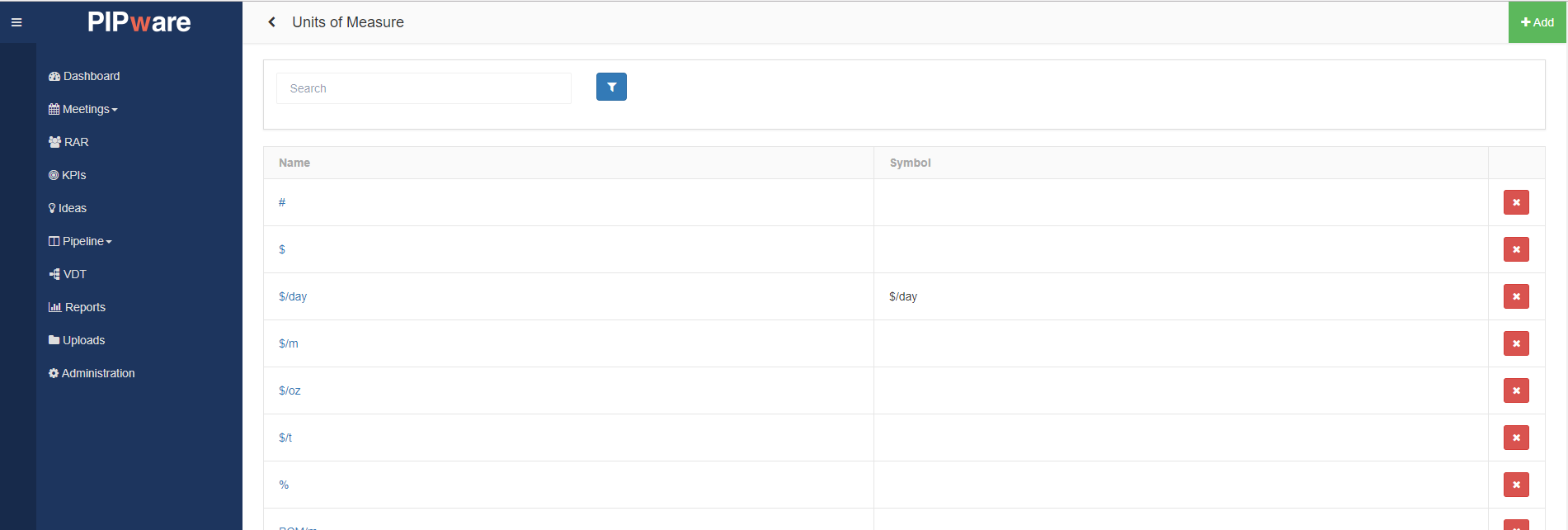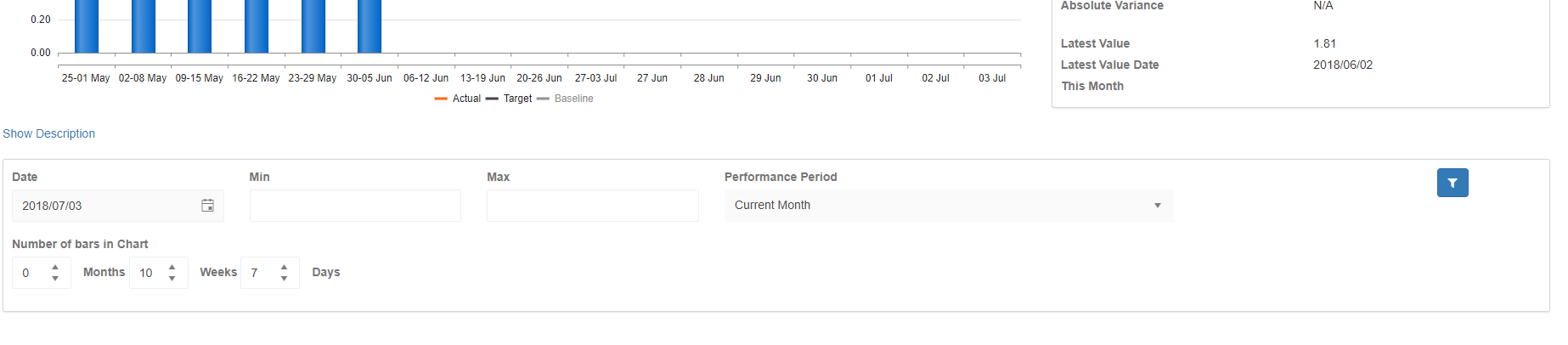Solution:

This usually occurs when the KPI is assigned a unit of measure that has a display factor of 0, hence the calculation becomes value*displayfactor = value*0 = 0.

i.e. usually a Percentage measurement/ unit of measure will have a 100% display factor. So a value of 0.4568 becomes 45.86%.

To resolve this issue you will need to capture the correct display factor for the measurement type.

You will need to have the permission to edit the measurement types, Administration -> Units of Measure.Also, confirm that there are values that satisfy the specified min and max values of the filters below the chart.